﻿ 广东省经济增长影响因素探讨——基于柯布道格拉斯生产函数

# 广东省经济增长影响因素探讨——基于柯布道格拉斯生产函数Discussion on Influencing Factors of Economic Growth in Guangdong Province—Based on Cobb Douglas Product Function

Abstract: Achieving stable economic growth is one of the objectives of monetary policy, and the Cobb Douglas product function can explain to some extent the contribution of factors such as labor, capital and energy to economic growth. This paper selects time series data such as labor volume, capital stock, fossil energy input and GDP in Guangdong Province from 2000 to 2019, and uses the Cobb Douglas product function to fit, and discusses the factors affecting the economic growth of Guangdong Province.

1. 引言

2. 文献综述

3. 数据来源及研究方法

3.1. 数据来源Table 1. Historical GDP of Guangdong Province and economic growth factor input table

3.2. 柯布道格拉斯函数原理介绍

$y=f\left(x\right)=f\left({x}_{1},{x}_{2},{x}_{3}\right)=A{x}_{1}^{\alpha }{x}_{2}^{\beta }{x}_{3}^{\gamma }$, $\theta =\alpha +\beta +\gamma$

$y=f\left(x\right)=f\left({x}_{1},{x}_{2},{x}_{3}\right)={\text{e}}^{g\left(t\right)}{x}_{1}^{\alpha }{x}_{2}^{\beta }{x}_{3}^{\gamma }$, $\theta =\alpha +\beta +\gamma$

3.3. 拟合过程及结果Table 2. Summary table of various factor coefficients

$y={\text{e}}^{0.0253{x}^{3}-0.076{x}^{2}+0.556x+8.8425}{x}_{1}^{0.5038}{x}_{2}^{0.2029}{x}_{3}^{0.2932}$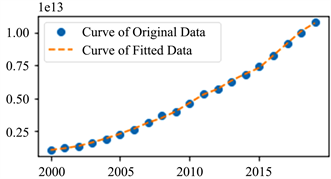Figure 1. Guangdong Province GDP forecast map

4. 拟合结果分析

4.1. 基于边际弹性分析广东省经济增长驱动力

${e}_{1}=\frac{dy}{y}/\frac{d{x}_{1}}{{x}_{1}}=\alpha$

${e}_{2}=\frac{dy}{y}/\frac{d{x}_{2}}{2}=\beta$

${e}_{3}=\frac{dy}{y}/\frac{d{x}_{3}}{3}=\gamma$

4.2. 基于边际产出和边际技术替代率探索广东未来发展新动力

$M{P}_{1}=\frac{\partial y}{\partial {x}_{1}}=\alpha A{x}_{1}^{\alpha -1}{x}_{2}^{\beta }{x}_{3}^{\gamma }=\frac{\alpha y}{{x}_{1}}$

$M{P}_{2}=\frac{\partial y}{\partial {x}_{2}}=\beta A{x}_{1}^{\alpha }{x}_{2}^{\beta -1}{x}_{3}^{\gamma }=\frac{\beta y}{{x}_{2}}$

$M{P}_{3}=\frac{\partial y}{\partial {x}_{3}}=\gamma A{x}_{1}^{\alpha }{x}_{2}^{\beta }{x}_{3}^{\gamma -1}=\frac{\gamma y}{{x}_{3}}$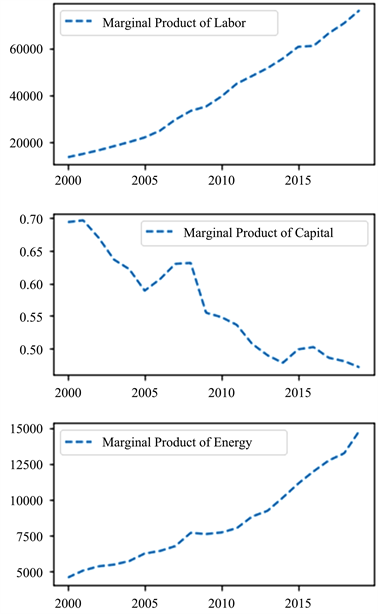Figure 2. A summary chart of the marginal product of economic factors in Guangdong Province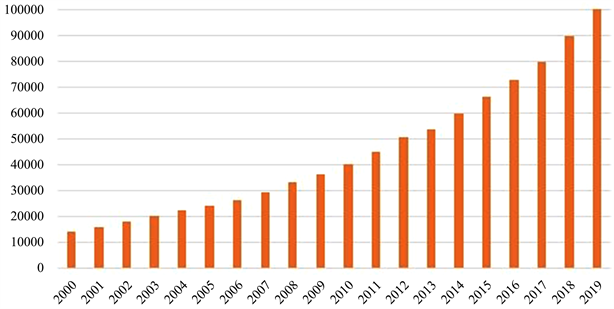Figure 3. Changes in annual average wages of urban employees in Guangdong Province

${\text{MRTS}}_{\text{1},\text{2}}=\frac{M{P}_{1}}{M{P}_{2}}=\frac{\frac{\alpha y}{{x}_{1}}}{\frac{\beta y}{{x}_{2}}}=\frac{\alpha }{\beta }\left(\frac{{x}_{2}}{{x}_{1}}\right)$

${\text{MRTS}}_{\text{1},\text{3}}=\frac{M{P}_{1}}{M{P}_{3}}=\frac{\frac{\alpha y}{{x}_{1}}}{\frac{\gamma y}{{x}_{3}}}=\frac{\alpha }{\gamma }\left(\frac{{x}_{3}}{{x}_{1}}\right)$

${\text{MRTS}}_{\text{2},\text{3}}=\frac{M{P}_{2}}{M{P}_{3}}=\frac{\frac{\beta y}{{x}_{2}}}{\frac{\gamma y}{{x}_{3}}}=\frac{\beta }{\gamma }\left(\frac{{x}_{3}}{{x}_{2}}\right)$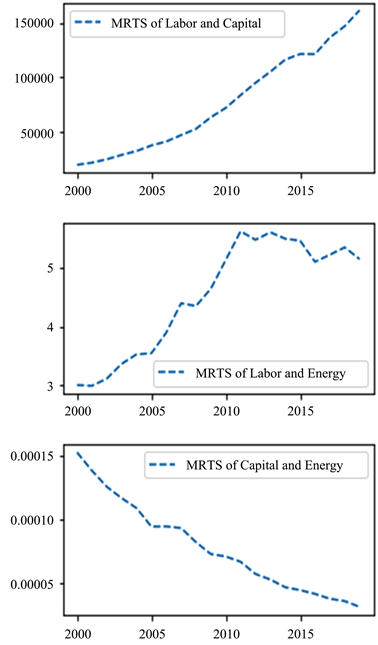Figure 4. Marginal technology substitution rate between the three major factors of productionFigure 5. Changes in the proportion of different energy consumption in Guangdong from 2000 to 2019

4.3. 全要素生产率

$y=f\left(x\right)=f\left({x}_{1},{x}_{2},{x}_{3}\right)=A{x}_{1}^{\alpha }{x}_{2}^{\beta }{x}_{3}^{\gamma }$

$dy=A\left(M{P}_{1}d{x}_{1}+M{P}_{2}d{x}_{2}+M{P}_{3}d{x}_{3}\right)$

$\frac{dy}{y}=A\left(\frac{M{P}_{1}}{y}d{x}_{1}+\frac{M{P}_{2}}{y}d{x}_{2}+\frac{M{P}_{3}}{y}d{x}_{3}\right)$

$\frac{M{P}_{1}}{y}=\frac{\alpha }{{x}_{1}}$, $\frac{M{P}_{2}}{y}=\frac{\beta }{{x}_{2}}$, $\frac{M{P}_{3}}{y}=\frac{\gamma }{{x}_{3}}$

$\frac{dy}{y}=A\left(\frac{\alpha }{{x}_{1}}d{x}_{1}+\frac{\beta }{{x}_{2}}d{x}_{2}+\frac{\gamma }{{x}_{3}}d{x}_{3}\right)$

$\frac{dy}{y}=A\left(\alpha \frac{d{x}_{1}}{{x}_{1}}+\beta \frac{d{x}_{2}}{{x}_{2}}+\gamma \frac{d{x}_{3}}{{x}_{3}}\right)$

$A=\frac{\frac{dy}{y}}{\alpha \frac{d{x}_{1}}{{x}_{1}}+\beta \frac{d{x}_{2}}{{x}_{2}}+\gamma \frac{d{x}_{3}}{{x}_{3}}}$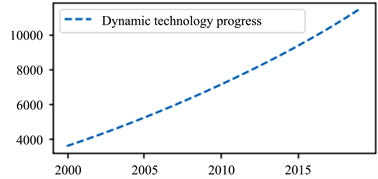Figure 6. Trend chart of total factor productivity from 2000 to 2019

5. 总结和政策建议

 王虹, 任宇杰. 陕西省影响经济增长的因素研究——基于柯布道格拉斯生产函数[J]. 商, 2015(20): 262.

 金伟娜, 张卓涵. 1993-2008年广东省经济增长的要素贡献分析——基于新柯布-道格拉斯生产函数[J]. 现代工业经济和信息化, 2012(8): 19-22.

 朱道才. 安徽省经济增长因素实证分析——基于1996-2007年数据[J]. 平顶山学院学报, 2009, 24(2): 17-23.

 骆娜, 黄文姗. 安徽省经济增长要素贡献率的实证分析——基于经济增长模型和柯布-道格拉斯函数的检验[J].南京航空航天大学学报(社会科学版), 2018, 20(3): 33-39.

 佘时飞. 经济增长理论文献综述[J]. 科技经济市场, 2009(8): 38-39.

 阎虎勤. Python财务数据分析(讲义) [M]. 厦门: 厦门国家会计学院, 2021.

 刘三林, 吴华维, 彭穗生. 人口红利与经济增长: 基于广东1987-2010年数据的分析[J]. 商业研究, 2013(3): 132-136.

 何杰锋. 广东经济增长的驱动因素: 1978-2012 [D]: [硕士学位论文]. 广州: 华南理工大学, 2014.

 李凤文. 引导贷款利率下降要多措并举[N]. 中国银行保险报, 2021-03-15(002).

 张炳君, 孙习武. 经济增长的全要素生产率研究[J]. 中国海洋大学学报(社会科学版), 2015(5): 73-78.

 唐未兵, 傅元海, 王展祥. 技术创新、技术引进与经济增长方式转变[J]. 经济研究, 2014, 49(7): 31-43.

 许长青. 人力资本、高等教育与区域经济增长——基于广东省的实证分析[J]. 高等工程教育研究, 2013(2): 90-96.

 李雪莉. 广东省能源消费与经济增长关系研究及能源预警分析[D]: [硕士学位论文]. 广州: 暨南大学, 2013.

Top